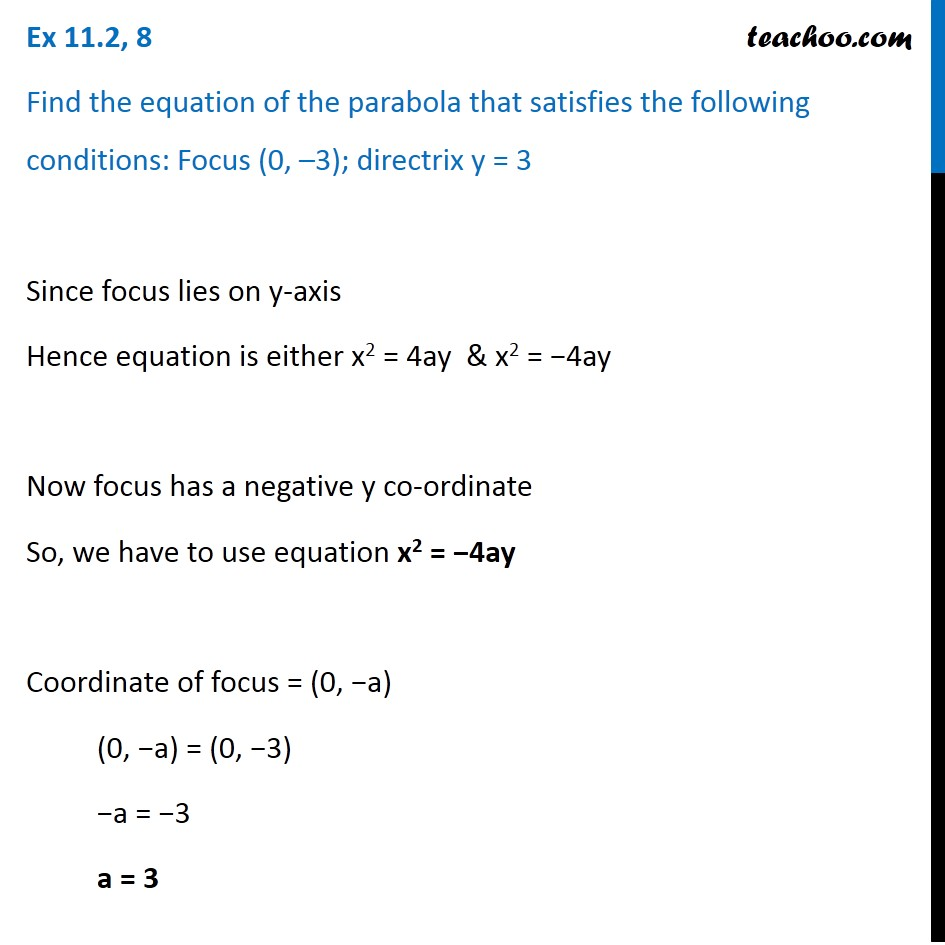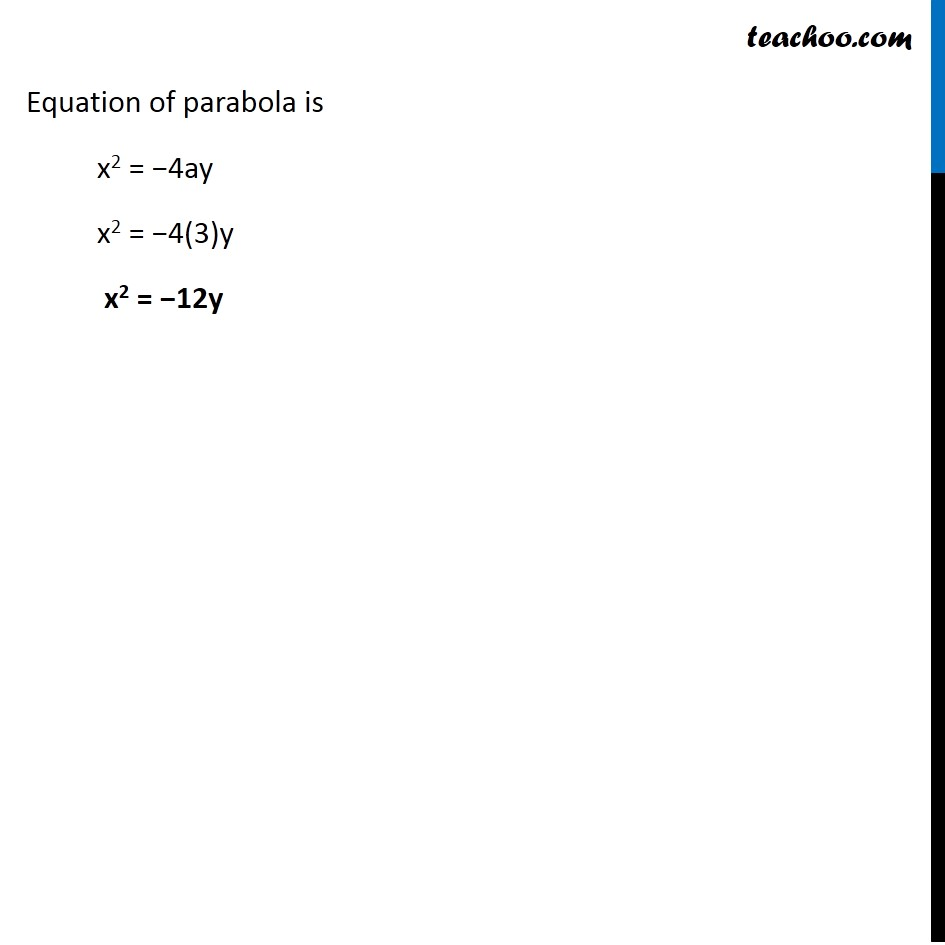Ex 10.2

Chapter 10 Class 11 Conic Sections
Serial order wiseLearn in your speed, with individual attention - Teachoo Maths 1-on-1 Class

### Transcript

Ex 10.2, 8 Find the equation of the parabola that satisfies the following conditions: Focus (0, –3); directrix y = 3 Since focus lies on y-axis Hence equation is either x2 = 4ay & x2 = −4ay Now focus has a negative y co-ordinate So, we have to use equation x2 = −4ay Coordinate of focus = (0, −a) (0, −a) = (0, −3) −a = −3 a = 3 Equation of parabola is x2 = −4ay x2 = −4(3)y x2 = −12y